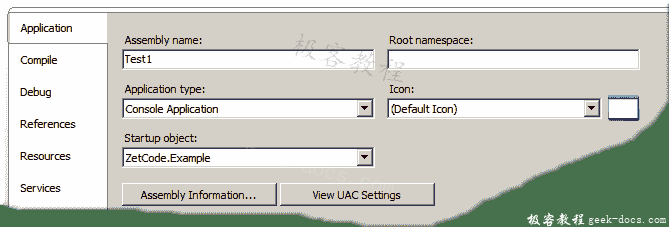# Visual Basic 组织代码

Visual Basic 组织代码，Visual Basic 语句被组织为块，模块，类和名称空间。 这有助于使代码更具可维护性和健壮性。 正确的代码组织可以防止在代码中出错。

Visual Basic 程序的基本构建块是：

• 部件
• 命名空间
• 模块
• 程序和功能
• 代码块
• 语句

## 基本例子

Option Strict On

Module Example

Sub Main()

Console.WriteLine("Simple example")

End Sub

End Module



Option Strict On

Public Class Example

Public Shared Sub Main()

Console.WriteLine("Simple example")

End Sub

End Class



## 命名空间

Option Strict On

NameSpace ZetCode

Module Example1

Public Dim x As Integer = 0

Sub Init()

x += 100
Console.WriteLine(x)

End Sub

End Module

End NameSpace



NameSpace ZetCode



Public Dim x As Integer = 0



Sub Init()
x += 100
Console.WriteLine(x)
End Sub



Option Strict On

NameSpace ZetCode

Module Example

Sub Main()

Init()
x += 100
Console.WriteLine(x)

End Sub

End Module

End NameSpace



NameSpace ZetCode



Init()
x += 100
Console.WriteLine(x)



$./samenamespace.exe 100 200  输出。 以下代码示例具有两个不同的名称空间。 我们使用Imports关键字从其他名称空间导入元素。 Option Strict On NameSpace MyMath Public Class Basic Public Shared PI As Double = 3.141592653589 Public Shared Function GetPi() As Double Return Me.PI End Function End Class End NameSpace  我们在MyMath名称空间中具有Math类的框架。 在 Basic 类中，我们定义PI常量和GetPi()方法。 Option Strict On Imports MyMath NameSpace ZetCode Public Class Example Public Shared Sub Main() Console.WriteLine(Basic.PI) Console.WriteLine(Basic.GetPi()) End Sub End Class End NameSpace  在此文件中，我们使用MyMath命名空间中的元素。 Imports MyMath  我们将元素从MyMath命名空间导入到我们的命名空间中。在 Visual Basic 2008 Express 版上，会自动创建一个根名称空间。 可以在项目属性的“应用”选项卡下找到。 删除根名称空间或将其包含在导入路径中。 例如，如果在那里有“测试”，则将行更改为 Imports Testing.MyMath Console.WriteLine(Basic.PI) Console.WriteLine(Basic.GetPi())  现在我们可以使用这些元素。 在我们的例子中，PI变量和GetPi()方法。 Option Strict On ' Imports MyMath NameSpace ZetCode Public Class Example Public Shared Sub Main() Console.WriteLine(MyMath.Basic.PI) Console.WriteLine(MyMath.Basic.GetPi()) End Sub End Class End NameSpace  请注意，我们不需要Imports关键字。 在示例中，将其注释掉。 我们可以通过使用元素的完全限定名称来使用其他命名空间中的元素。 ## 模块 模块用于组织代码并包装类似用途的变量，属性，事件和过程。 与类不同，模块不是类型。 可以在名称空间或文件中创建模块。 不能在另一个模块，类，结构，接口或块内创建模块。 模块中的所有成员都是隐式共享的。 模块具有“朋友”访问权限。 这意味着模块可在装配中的任何位置访问。 Option Strict On Module First Public x As Byte = 11 Public Sub FirstModule() Console.WriteLine("First module") End Sub End Module Module Second Public y As Byte = 22 Public Sub SecondModule() Console.WriteLine("Second module") End Sub End Module Module Example Sub Main() Console.WriteLine(x) Console.WriteLine(Second.y) FirstModule() SecondModule() End Sub End Module  我们定义了三个模块。 前两个模块具有变量和过程。 这些将在第三个模块中使用。 Module First Public x As Byte = 11 ... End Module  我们也可以在模块内部使用访问说明符。 这样，我们可以控制模块中元素的可访问性。 Console.WriteLine(x) Console.WriteLine(Second.y)  我们打印xy变量。 它们是Public，可以从其他模块访问。 我们可以使用模块名称来完全指定变量名称。 作用域是变量的可见性。 具有模块作用域的变量在声明了该模块的模块中可用。 Option Strict On Module Example Private x As Integer = 0 Sub Main() proc1() proc2() proc3() End Sub Sub proc1() Console.WriteLine(x) End Sub Sub proc2() x += 100 Console.WriteLine(x) End Sub Sub proc3() x += 100 Console.WriteLine(x) End Sub End Module  我们在模块内部有x变量。 该变量在所有三个过程中均可用。 Private x As Integer = 0  这是具有模块作用域的变量。 它在任何过程外声明。 Sub proc2() x += 100 Console.WriteLine(x) End Sub  proc2()过程中，我们增加了x变量并将其内容打印到控制台。 我们引用模块中定义的x变量。 $ ./modulescope.exe
0
100
200



## 过程

Option Strict On

Module Example

Dim x As Integer = 0

Sub Main()

Console.WriteLine(x)

proc1()
proc2()
proc3()

End Sub

Sub proc1()

Dim x As Integer
x += 100

Console.WriteLine(x)

End Sub

Sub proc2()

Dim x As Integer
x += 100
Console.WriteLine(x)

End Sub

Sub proc3()

Dim x As Integer
x += 100
Console.WriteLine(x)

End Sub

End Module



Sub proc1()
Dim x As Integer
x += 100
Console.WriteLine(x)
End Sub



proc1()过程创建一个本地x变量。 此变量遮盖了，该变量在模块作用域中声明。

\$ ./procedurescope.exe
0
100
100
100



## 块作用域

Option Strict On

Module Example

Sub Main()

If True

Console.WriteLine("Inside If block")

Dim x As Integer = 0
Console.WriteLine(x)

x += 500
Console.WriteLine(x)

End If

Console.WriteLine("Outside If block")

Rem Will not compile
Rem Console.WriteLine(x)

End Sub

End Module



Rem Will not compile
Rem Console.WriteLine(x)



• 回顶